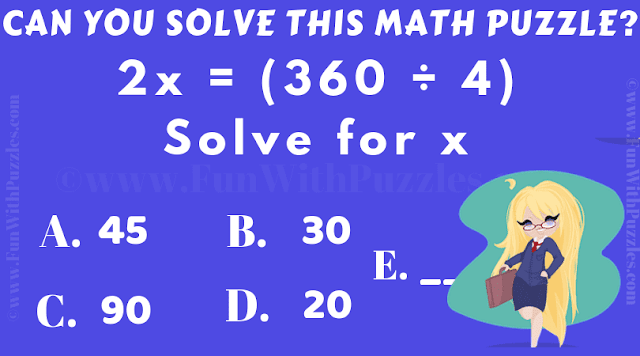This is an easy maths puzzle for kids. Solving this maths puzzle will test your mathematical skills and your quickness to solve a given problem. This is an easy maths puzzle which needs to solved quickly. Lets us see if you can solve this maths puzzle in less than a minute?Can you solve this Maths Puzzle?
The answer to this "Easy Maths Puzzle for Kids", can be viewed by clicking on the answer button.